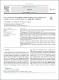## Use of a system of differential equations to analyze the functioning of a catalytic bio macromolecule under non equilibrium conditions2019
Barabash, Y.
Serdenko, T.
Knox, P.
Golub, Alexander
##### Abstract
The aim of the work is to analyze the response of a biomolecule to an external influence based on the study of its hidden states by identifying differential equations with constant coefficients. The relevance of the work lies in the fact that often the main reaction of an object to an external action can be represented as a sum of various exponential functions with a common starting point and a material balance equation. In this case, the response of an object to an external action corresponds to a system of differential equations with constant coefficients. This character of the main reaction may be due to the influence of the hidden properties of the object, which play the role of regulatory parameters. The problem is that the hidden factors and the system of differential equations are not identified. As an object, isolated reaction centers (RC) of the bacteria Rhodobacter sphaeroides, which possess the above properties, has used. Their structure is well studied. As result of studying of photo excitation processes of the reaction center has shown that electron transfer kinetics (the main reaction) can be approximated by three normalized exponential functions. Program was developed to identify for four differential equations of electron transfer and the balance equation, the behavior of hidden states of the reaction center. It was concluded that time the dependence the probability density of finding an electron in different conformational states of the reaction center characterizes the space-time changes in the structure of the reaction center.
##### Keywords
Biophysics, Electron-conformational sub states, Structural self-regulation, Reaction centers of Rhodobacter sphaeroides, Electron transport, Mathematical model, article
##### Citation
Use of a system of differential equations to analyze the functioning of a catalytic bio macromolecule under non equilibrium conditions [electronic resource] / Barabash Y. M., Serdenko T. V., Knox P. P., Golub A. A. // Heliyon. - 2019. - Vol. 5, Issue 7. - Article number e02108.